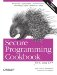# Finding the nth Instance of a Substring

Problem

Given two strings source and pattern, you want to find the nth occurrence of pattern in source.

Solution

Use the find member function to locate successive instances of the substring you are looking for. Example 4-17 contains a simple nthSubstr function.

Example 4-17. Locate the nth version of a substring

```#include
#include

using namespace std;

int nthSubstr(int n, const string& s,
const string& p) {
string::size_type i = s.find(p); // Find the first occurrence

int j;
for (j = 1; j < n && i != string::npos; ++j)
i = s.find(p, i+1); // Find the next occurrence

if (j == n)
return(i);
else
return(-1);
}

int main( ) {
string s = "the wind, the sea, the sky, the trees";
string p = "the";

cout << nthSubstr(1, s, p) << '
';
cout << nthSubstr(2, s, p) << '
';
cout << nthSubstr(5, s, p) << '
';
}```

Discussion

There are a couple of improvements you can make to nthSubstr as it is presented in Example 4-17. First, you can make it generic by making it a function template instead of an ordinary function. Second, you can add a parameter to account for substrings that may or may not overlap with themselves. By "overlap," I mean that the beginning of the string matches part of the end of the same string, as in the word "abracadabra," where the last four characters are the same as the first four. Example 4-18 demonstrates this.

Example 4-18. An improved version of nthSubstr

```#include
#include

using namespace std;

template
int nthSubstrg(int n, const basic_string& s,
const basic_string& p,
bool repeats = false) {
string::size_type i = s.find(p);
string::size_type adv = (repeats) ? 1 : p.length( );

int j;
for (j = 1; j < n && i != basic_string::npos; ++j)

if (j == n)
return(i);
else
return(-1);
}

int main( ) {
string s = "AGATGCCATATATATACGATATCCTTA";
string p = "ATAT";

cout << p << " as non-repeating occurs at "
<< nthSubstrg(3, s, p) << '
';
cout << p << " as repeating occurs at "
<< nthSubstrg(3, s, p, true) << '
';
}```

The output for the strings in Example 4-18 is as follows:

```ATAT as non-repeating occurs at 18
ATAT as repeating occurs at 11```

Recipe 4.9Secure Programming Cookbook for C and C++: Recipes for Cryptography, Authentication, Input Validation & More
ISBN: 0596003943
EAN: 2147483647
Year: 2006
Pages: 241